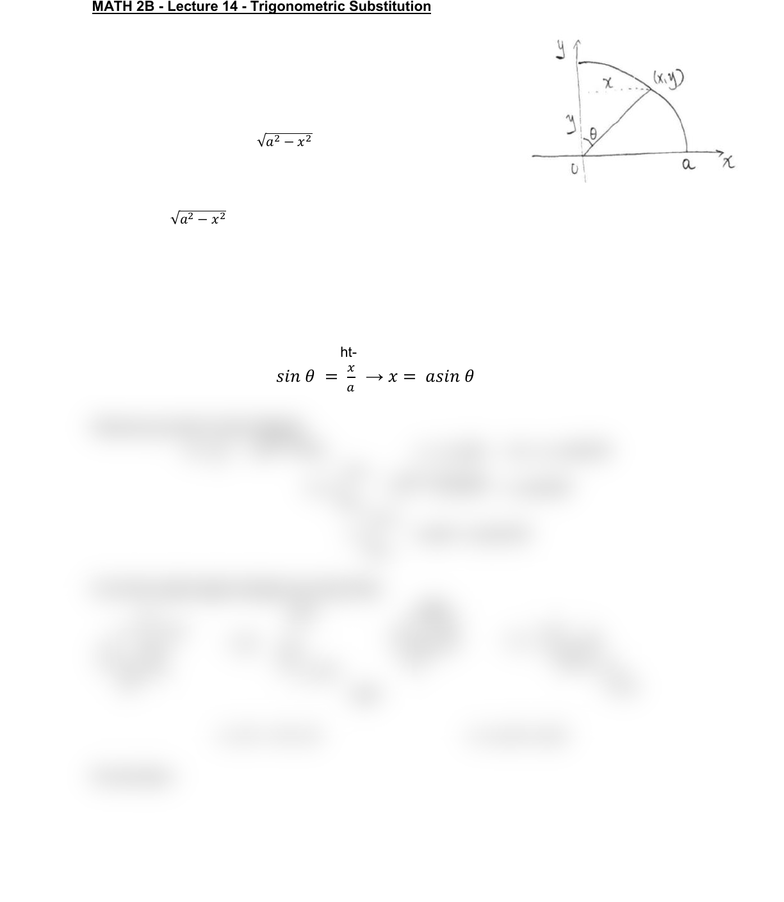Class Notes (1,100,000)
US (460,000)
UC-Irvine (10,000)
MATH (1,000)
MATH 2B (600)
Lecture 14

# MATH 2B Lecture Notes - Lecture 14: Trigonometric SubstitutionPremium

Department
Mathematics
Course Code
MATH 2B
Professor
ERJAEE, G.
Lecture
14

This preview shows half of the first page. to view the full 2 pages of the document.02/06/2019
MATH 2B - Lecture 14 - Trigonometric Substitution
We begin by considering the area of a quarter circle with radius a:
First we know the curve representing a circle is  
Then we have  
Take the square-root on both sides and only keep the
positive part, we have:
   (range for the
quarter)
So the area of a quarter circle can be written as  

Now, we will learn how to use trig. Sub to solve the integral
Using trigonometric substitution
We first pick up a point on the curve and denote it as (x,y)
Connecting this point to the center, we obtain a right-angle triangle
The long side is the radius a, two legs are x,y
Due to the property of right-angle trigonometry, we have:


Now we go back to the integral
   

 
From the right-angle triangle we know that
and
 
So we have: